• +91 9971497814
• info@interviewmaterial.com

# RD Chapter 4- Triangles Ex-4.7 Interview Questions Answers

### Related Subjects

Question 1 : If the sides of a triangle are 3 cm, 4 cm and 6 cm long, determine whether the triangle is a right-angled triangle. (C.B.S.E. 1992)

We know that if the square of the hypotenuse (longest side) is equal to the sum of squares of other two sides then it is right triangle
Now the sides of a triangle are 3 cm, 4 cm and 6 cm
(Longest side)² = (6)² = 36
and sum of two smaller sides = (3)² + (4)² = 9 + 16 = 25
36 ≠ 25
It is not a right-angled triangle

Question 2 :
The sides of certain triangles are given below. Determine which of them are right triangles :
(i) a = 1 cm, b = 24 cm and c = 25 cm
(ii) a = 9 cm, b = 16 cm and c = 18 cm
(iii) a = 1.6 cm, b = 3.8 cm and c = 4 cm
(iv) a = 8 cm, b = 10 cm and c = 6 cm (C.B.S.E. 1992)

We know that if the square of hypotenuse is equal to the sum of squares of other two sides, then it is a right triangle
(i) Sides of a triangle are a = 7 cm, b = 5.24 cm and c = 25 cm
(Longest side)² = (25)² = 625
Sum of square of shorter sides = (7)² + (24)² = 49 + 576 = 625
625 = 625
This is right triangle
(ii) Sides of the triangle are a = 9 cm, b = 16 cm, c = 18 cm
(Longest side)² = (18)² = 324
and sum of squares of shorter sides = (9)² + (16)² = 81 + 256 = 337
324 ≠ 337
It is not a right-angled triangle
(iii) Sides of the triangle are a = 1.6 cm, 6 = 3.8 cm, c = 4 cm
(Longest side)² = (4)² =16
Sum of squares of shorter two sides + (1.6)² + (3.8)² = 2.56 + 14.44 = 17.00
16 ≠ 17
It is not a right triangle
(iv) Sides of the triangle are a = 8 cm, b = 10 cm, c = 6 cm
(Longest side)² = (10)² = 100
Sum of squares of shorter sides = (8)² + (6)² = 64 + 36 = 100
100 = 100
It is a right triangle

Question 3 : A man goes 15 metres due west and then 8 metres due north. How far is he from the starting point ?

Let a man starts from O, the starting point to west 15 m at A and then from A, 8 m due north at B
Join OB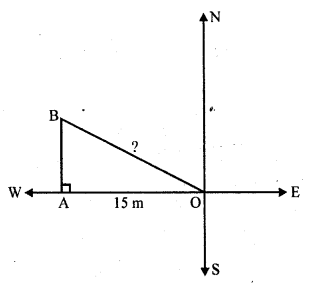Now in right ∆OAB
OB² = OA² + AB² (Pythagoras Theorem)
OB² = (15)² + (8)² = 225 + 64 = 289 = (17)²
OB = 17
The man is 17 m away from the starting point

Question 4 : A ladder 17 m long reaches a window of a building 15 m above the ground. Find the distance of the foot of the ladder from the building.

Length of ladder = 17 m
Height of window = 15 m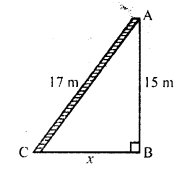Let the distance of the foot of ladder from the building = x
Using Pythagoras Theorem
AC² = AB² + BC²
=> (17)² = (15)² + x²
=> 289 = 225 + x²
=> x² = 289 – 225
=> x² = 64 = (8)²
x = 8
Distance of the foot of the ladder from the building = 8m

Question 5 : Two poles of heights 6 m and 11 m stand on a plane ground. If the distance between their feet is 12 m, find the distance between their tops. (C.B.S.E. 1996C, 2002C)

Two poles AB and CD which are 6 m and 11 m long respectively are standing oh the ground 12 m apart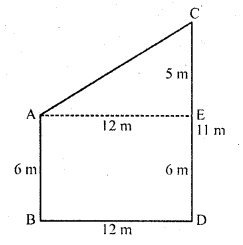Draw AE || BD so that AE = BD = 12 m and ED = AB = 6 m
Then CE = CD – ED = 11 – 6 = 5 m
Now in right ∆ACE
Using Pythagoras Theorem,
AC² = AE² + EC² = (12)² + (5)² = 144 + 25 = 169 = (13)²
AC = 13
Distance between their tops = 13 m

Question 6 : In an isosceles triangle ABC, AB = AC = 25 cm, BC = 14 cm. Calculate the altitude from A on BC. (C.B.S.E. 1994)

∆ABC is an isosceles triangle in which AB = AC = 25 cm .
AD ⊥ BC BC = 14 cm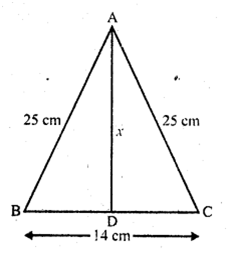Perpendicular AD bisects the base i.e . BD = DC = 7 cm
In right ∆ABD,
AB² = AD² + BD² (Pythagoras Theorem)
=> (25)² = AD² + (7)²
=> 625 = AD² + 49
=> AD² = 625 – 49
=> AD² = 576 = (24)²

Question 7 : The foot of a ladder is 6 m away from a wall and its top reaches a window 8 m above the ground. If the ladder is shifted in such a way that its foot is 8 m away from the wall, to what height does its tip reach ?

In first case,
The foot of the ladder are 6 m away from the wall and its top reaches window 8 m high
Let AC be ladder and BC = 6 m, AB = 8 m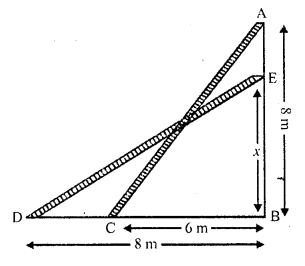Now in right ∆ABC,
Using Pythagoras Theorem
AC² = BC² + AB² = (6)² + (8)² = 36 + 64 = 100 = (10)²
AC = 10 m
In second case,
ED = AC = 10 m
BD = 8 m, let ED = x
ED² = BD² + EB²
=> (10)² = (8)² + x²
=> 100 = 64 + x²
=> x² = 100 – 64 = 36 = (6)²
x = 6
Height of the ladder on the wall = 6 m

Question 8 : Two poles of height 9 m and 14 m stand on a plane ground. If the distance between their feet is 12 m, find the distance between their tops.

Let CD and AB be two poles which are 12 m apart
AB = 14 m, CD = 9 m and BD = 12 m
From C, draw CE || DB
CB = DB = 12 m
EB = CD = 9 m
and AE = 14 – 9 = 5 m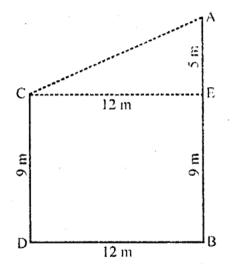Now in right ∆ACE,
AC² = AE² + CE² (Pythagoras Theorem)
= (5)² + (12)²
= 25 + 144 = 169 = (13)²
AC = 13
Distance between their tops = 13 m

Question 9 : Using Pythagoras theorem, determine the length of AD in terms of b and c shown in the figure. (C.B.S.E. 1997C)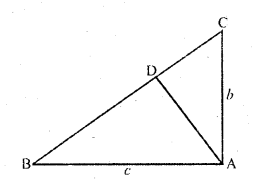In right ∆ABC, ∠A = 90°
AB = c, AC = b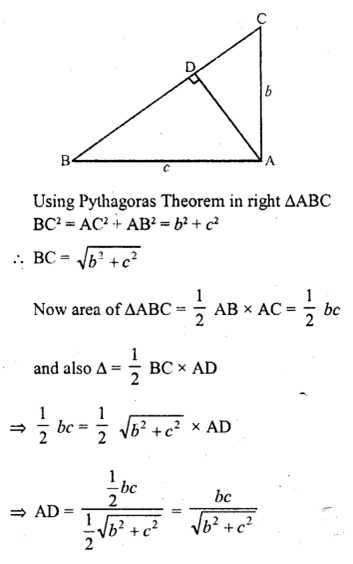Question 10 : A triangle has sides 5 cm, 12 cm and 13 cm. Find the length to one decimal place, of the perpendicular from the opposite vertex to the side whose length is 13 cm. (C.B.S.E. 1992C)

A triangle has sides 5 cm, 12 cm and 13 cm
(Longest side)² = (13)² = 169
Sum of squares of shorter sides = (5)² + (12)² = 25 + 144= 169
169 = 169
It is a right triangle whose hypotenuse is 13 cm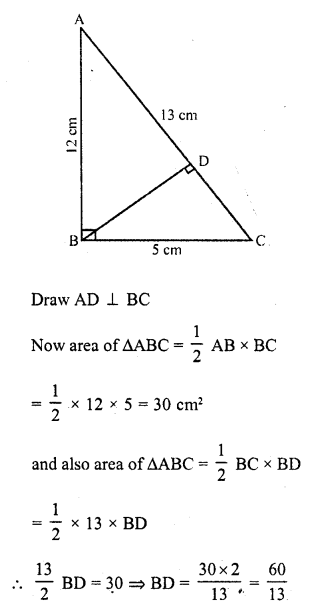Todays Deals### RD Chapter 4- Triangles Ex-4.7 Contributorskrishan

Name:
Email:

# Latest News# 9000 interview questions in different categories